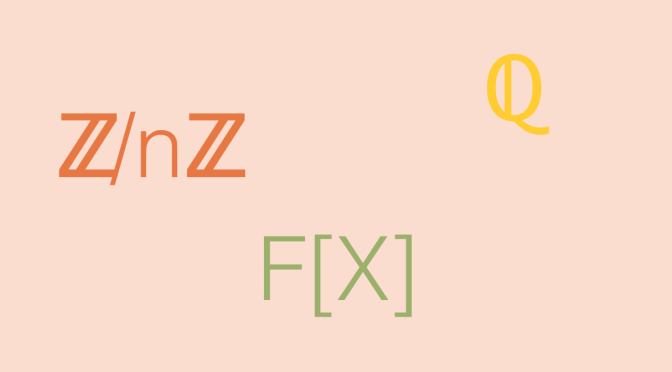# A module without a basis

Let’s start by recalling some background about modules.

Suppose that $$R$$ is a ring and $$1_R$$ is its multiplicative identity. A left $$R$$-module $$M$$ consists of an abelian group $$(M, +)$$ and an operation $$R \times M \rightarrow M$$ such that for all $$r, s \in R$$ and $$x, y \in M$$, we have:

1. $$r \cdot (x+y)= r \cdot x + r \cdot y$$ ($$\cdot$$ is left-distributive over $$+$$)
2. $$(r +s) \cdot x= r \cdot x + s \cdot x$$ ($$\cdot$$ is right-distributive over $$+$$)
3. $$(rs) \cdot x= r \cdot (s \cdot x)$$
4. $$1_R \cdot x= x$$

$$+$$ is the symbol for addition in both $$R$$ and $$M$$.
If $$K$$ is a field, $$M$$ is $$K$$-vector space. It is well known that a vector space $$V$$ is having a basis, i.e. a subset of linearly independent vectors that spans $$V$$.
Unlike for a vector space, a module doesn’t always have a basis.

## The module $$\mathbb{Z}/n\mathbb{Z}$$ of integers modulo $$n$$

Consider an integer $$n \ge 2$$ and the module $$M$$ of the integers modulo $$n$$ denoted $$\mathbb{Z}/n\mathbb{Z}$$ over the ring of the integers $$\mathbb{Z}$$. For all $$x \in M$$ we have $$n \cdot x = 0$$. So a subset of $$M$$ containing a non-zero element is linearly dependent. Therefore every non empty subset of $$M$$ is also linearly dependent and cannot be a basis. As the linear span of the empty subset of $$M$$ is defined to be $$\{0\}$$ (because by convention a linear combination of no vectors sums to $$0$$), $$M$$ has no basis.

$$\mathbb{Z}/n\mathbb{Z}$$ is having torsion. But there also exist torsion-free modules not having a basis as we prove in next paragraph. Let’s recall that a torsion-free module is a module over a ring such that $$0$$ is the only element annihilated by a regular element (non zero-divisor) of the ring.

## The module $$\mathbb{Q}$$ of the rational numbers over the integers

$$\mathbb{Q}$$ is a module over the ring of the integers $$\mathbb{Z}$$. $$\mathbb{Q}$$ is torsion-free. As for the previous example, the empty subset is not a basis because $$\mathbb{Q} \neq \{0\}$$.

### A subset with a single element does not span $$\mathbb{Q}$$

We take $$r = \frac{p}{q} \in \mathbb{Q}$$ where $$p,q$$ are integers. For any integer $$m \neq 0$$, we have $$\vert mr \vert > \vert \frac{1}{2q} \vert$$. So $$\frac{1}{2q} \notin \mbox{Span}(\{r\})$$ and $$\{r\}$$ cannot be a basis.

## A subset with two elements or more is dependent

We take a subset $$S \subset \mathbb{Q}$$ containing at least two elements $$r_1=\frac{p_1}{q_1},r_2=\frac{p_2}{q_2}$$. As $$(q_1 p_2) r_1- (q_2 p_1) r_2 = 0$$, $$S$$ is a dependent subset.

Finally, whatever is the cardinal of a subset $$S \subset \mathbb{Q}$$, it cannot be a basis of $$\mathbb{Q}$$ as a module over the integers.

## The module $$F[X]$$ over the ring $$F^\prime[X]$$

Let $$F[X]$$ be the ring of polynomials over a field $$F$$ and $$F^\prime[X]$$ be the ring of all polynomials of $$F[X]$$ that have coefficient of $$X$$ equal to $$0$$. Then $$F[X]$$ is an $$F^\prime[X]$$-module. $$F[X]$$ is finitely generated by the generating subset $$T=\{1,X\}$$ as any polynomial $$P(X)=a + bX + X^2 Q(X) \in F[X]$$ can be written $$(a+X^2Q(X)) \cdot 1+ b \cdot X$$. But $$T$$ is not an independent subset as follows from the relation $$X^3 \cdot 1 – X^2 \cdot X=0$$.

$$F[X]$$ is also torsion-free because it is an integral domain.

Let suppose that a subset $$S \subset F[X]$$ is a basis. $$S$$ cannot have a single element $$P$$ since if $$\deg(P)>0$$, the constant polynomials cannot be generated whereas if $$\deg(P)=0$$ that’s the polynomials having coefficient of $$X$$ different than $$0$$ that cannot be generated. But if $$S$$ contains two distinct polynomials $$P_1, P_2$$ then $$S$$ is a dependent subset as $$(X^2 P_2) \cdot P_1 – (X^2 P_1) \cdot P_2=0$$.